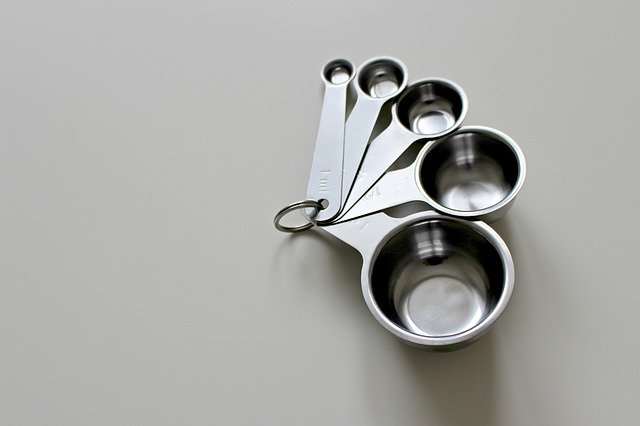# Cooking Conversion ChartOven temperatures

140C = 275F = Gas Mark 1

150C = 300F = Gas Mark 2

160C = 325F = Gas Mark 3

180C = 350F = Gas Mark 4

190C = 375F = Gas Mark 5

200C = 400F = Gas Mark 6

210C = 425F = Gas Mark 7

220C = 450F = Gas Mark 8

240C = 475F = Gas Mark 9

Liquid

1 tsp = 5ml

1 tbsp = 15ml

1 pint = 600ml

Weight

30g = 1oz

450g = 1lb

Cake tins

18cm = 7in

20cm = 8in

23cm = 9in

25cm = 10in

LET’S CONNECT!You can also subscribe to Foodie Explorers to keep up to date